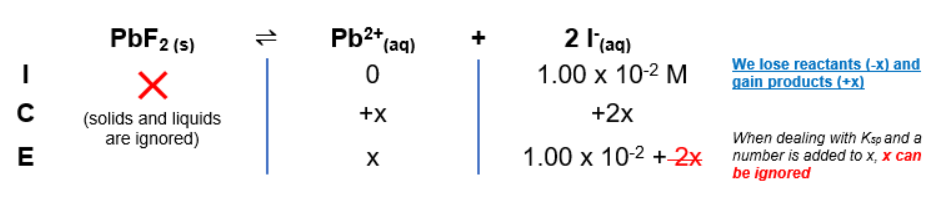# Problem: What concentration of the lead ion, Pb2+ , must be exceeded to precipitate PbF2 from a solution that is 1.00?10?2 M in the fluoride ion, F? ? Ksp for lead(II) fluoride is 3.3?10?8 .

###### FREE Expert Solution

For this problem, we’re being asked to calculate the concentration of Pb2+ needed in order to precipitate PbF2 from a 1.00 x 10-2 M Fsolution.

Dissociation reaction: PbF2(s)  Pb2+(aq) + 2F(aq)

Step 1: ICE ChartStep 2: Ksp expression

$\overline{){{\mathbf{K}}}_{{\mathbf{sp}}}{\mathbf{=}}\frac{\mathbf{products}}{\overline{)\mathbf{reactants}}}{\mathbf{=}}\mathbf{\left[}{\mathbf{Pb}}^{\mathbf{2}\mathbf{+}}\mathbf{\right]}{\mathbf{\left[}{\mathbf{F}}^{\mathbf{-}}\mathbf{\right]}}^{{\mathbf{2}}}}$

Note that each concentration is raised by the stoichiometric coefficient

84% (440 ratings)###### Problem Details

What concentration of the lead ion, Pb2+ , must be exceeded to precipitate PbF2 from a solution that is 1.00?10?2 M in the fluoride ion, F? ? Ksp for lead(II) fluoride is 3.3?10?8 .